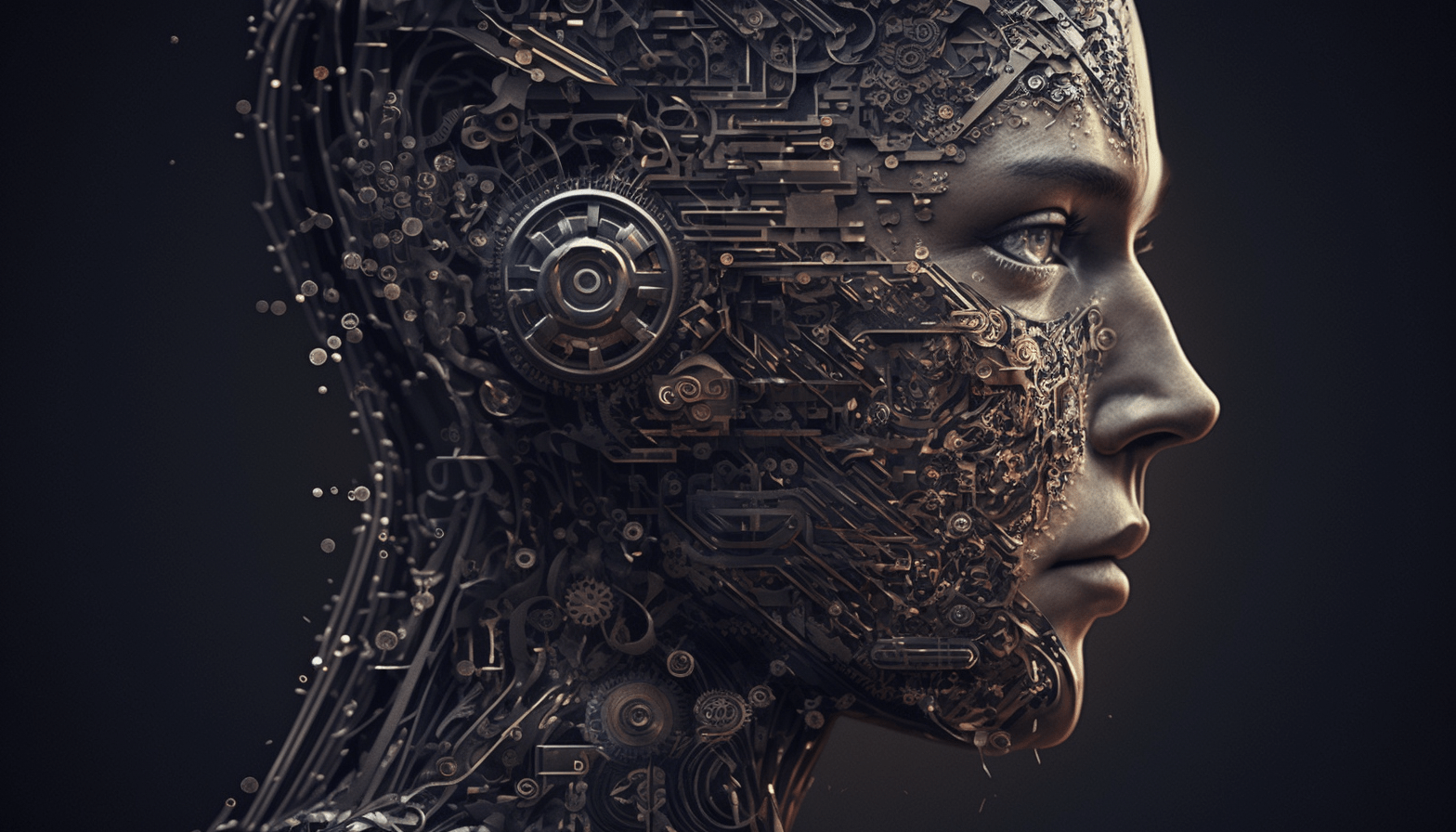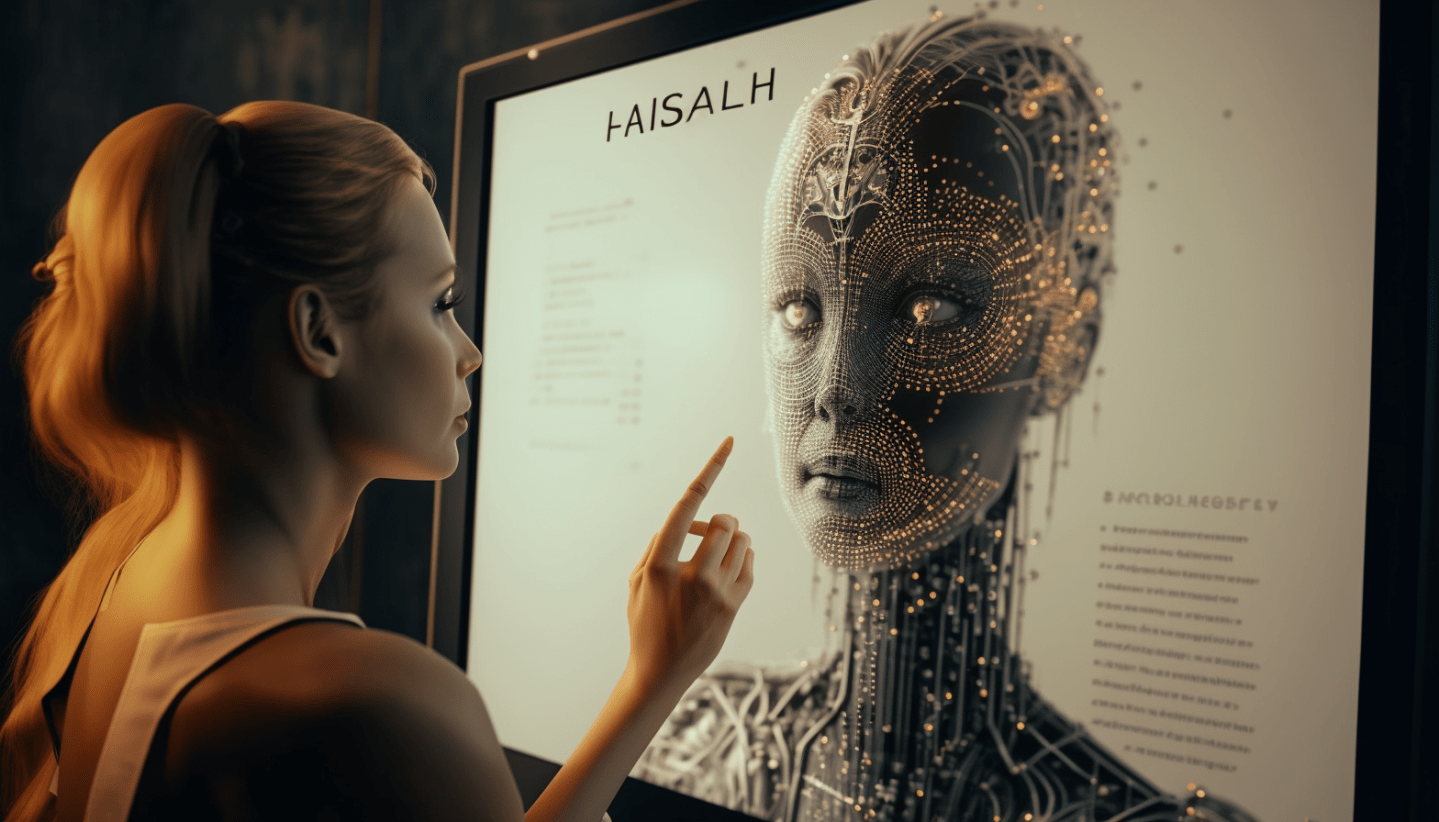# Artikler om AI

### Choose category

A.I. and Mathematical Optimization: A striking Synergy

">### A.I. and Mathematical Optimization: A striking Synergy

Artificial intelligence sounds sexy and we love the “Optimal” part of “Mathematical Optimization”. However, as we get down to earth and dig a little deeper we find assumptions and challenges making it hard to apply either technology in the real world. What if we put the two together, would we double...

A.I. and Mathematical Optimization: What's the difference?

">### A.I. and Mathematical Optimization: What's the difference?

The term Mathematical Optimization (MO) isn’t sexy or sci-fi at all, and unlike the term “AI” is more likely to give you nightmares about highschool algebra rather than dreams about sentient robots. However, we do like the sound of making things “optimal”, so let’s dig a little deeper.

">